# NB-IoT NPDSCH Block Error Rate Simulation

This example shows how LTE Toolbox™ can be used to create a NB-IoT Narrowband Physical Downlink Shared Channel (NPDSCH) Block Error Rate (BLER) simulation under frequency-selective fading and Additive White Gaussian Noise (AWGN) channel.

### Introduction

3GPP Release 13 of LTE started to add support for Narrowband IoT applications. Release 13 defines a single NB-IoT UE Category, namely Cat-NB1, and Release 14 adds Cat-NB2 which allows for larger transport block sizes. This example focuses on Release 13 NB-IoT.

The example generates a NB-IoT NPDSCH BLER curve for a number of SNR points and transmission parameters. NPSS and NSSS are transmitted in appropriate subframes and the NPSS is used for practical timing synchronization. NPSS and NSSS subframes are not used for NPDSCH transmission. The NRS is transmitted in NPDSCH subframes and is used for practical channel estimation. NPBCH transmission gaps are not considered in this example.

### Simulation Configuration

The simulation length is 4 DL-SCH transport blocks for a number of SNR points. A larger number of numTrBlks should be used to produce meaningful throughput results. SNR can be an array of values or a scalar. The simulation is performed over different repetition values to compare the performance improvement with repetitions.

numTrBlks = 4; % Number of simulated transport blocks SNRdB = -32:4:0; % SNR range in dB ireps = [0 5 9]; % Range of reps simulated 

### Setup Higher Layer Parameters

Setup the following higher layer parameters which are used to configure the NPDSCH in the next section:

• The variable NPDSCHDataType indicates whether the NPDSCH is carrying the SystemInformationBlockType1-NB (SIB1-NB) or not, and whether the NPDSCH is carrying the broadcast control channel (BCCH) or not. The allowed values of NPDSCHDataType are 'SIB1NB', 'BCCHNotSIB1NB' and 'NotBCCH'. Note that SIB1-NB belongs to the BCCH.

• The number of NPDSCH repetitions and the transport block size (TBS) are affected by whether NPDSCH is carrying SIB1-NB or not (see 3GPP TS 36.213 16.4.1.3 and 16.4.1.5 [ 2 ]). NPDSCHDataType set to 'SIB1NB' indicates that the NPDSCH is carrying SIB1-NB; NPDSCHDataType set to either 'BCCHNotSIB1NB' or 'NotBCCH' indicates that the NPDSCH is not carrying SIB1-NB.

• The NPDSCH repetition pattern and the scrambling sequence generation is affected by whether NPDSCH is carrying BCCH or not (see 3GPP TS 36.211 10.2.3 [ 1 ]). NPDSCHDataType set to either 'SIB1NB' or 'BCCHNotSIB1NB' indicates that the NPDSCH is carrying BCCH; NPDSCHDataType set to 'NotBCCH' indicates that the NPDSCH is not carrying BCCH.

NPDSCHDataType = 'NotBCCH'; % The allowed values are 'SIB1NB', 'BCCHNotSIB1NB' or 'NotBCCH' 
• The variable ISF configures the number of subframes for a NPDSCH according to 3GPP TS 36.213 Table 16.4.1.3-1 [ 2 ]. Valid values for ISF are 0...7.

When the NPDSCH carries the SIB1-NB:

• The variable SchedulingInfoSIB1 configures the number of NPDSCH repetitions according to 3GPP TS 36.213 Table 16.4.1.3-3 and the TBS according to Table 16.4.1.5.2-1 [ 2 ]. Valid values for SchedulingInfoSIB1 are 0...11.

When the NPDSCH does not carry the SIB1-NB:

• The variable IRep configures the number of NPDSCH repetitions according to 3GPP TS 36.213 Table 16.4.1.3-2 [ 2 ]. Valid values for IRep are 0...15.

• The variable IMCS together with IRep configure the TBS according to 3GPP TS 36.213 Table 16.4.1.5.1-1 [ 2 ]. Valid values for IMCS are 0...13.

ISF = 0; % Resource assignment field in DCI (DCI format N1 or N2) SchedulingInfoSIB1 = 0; % Scheduling information field in MasterInformationBlock-NB (MIB-NB) IMCS = 4; % Modulation and coding scheme field in DCI (DCI format N1 or N2) 

### eNB Configuration

Configure the starting frame and subframe numbers (enb.NFrame and enb.NSubframe) in the simulation for each SNR point, the narrowband physical cell ID enb.NNCellID, the number of NRS antenna ports (enb.NBRefP, one antenna port indicates port 2000 is used, two antenna ports indicates port 2000 and port 2001 are used), the NB-IoT operation mode enb.OperationMode which can be any value as follows:

• 'Standalone': NB-IoT carrier deployed outside the LTE spectrum, e.g. the spectrum used for GSM or satellite communications

• 'Guardband': NB-IoT carrier deployed in the guardband between two LTE carriers

• 'Inband-SamePCI': NB-IoT carrier deployed in resource blocks of a LTE carrier, with enb.NBRefP the same as the number of CRS ports enb.CellRefP

• 'Inband-DifferentPCI': NB-IoT carrier deployed in resource blocks of a LTE carrier, with enb.NBRefP different as enb.CellRefP

enb.CellRefP is configured when the operation mode is 'Inband-DifferentPCI'. The starting OFDM symbol index in a subframe for NPDSCH is configured using enb.ControlRegionSize, when the values of NPDSCHDataType and enb.OperationMode satisfy the following conditions:

• NPDSCHDataType is either 'BCCHNotSIB1NB' or 'NotBCCH'

• enb.OperationMode is either 'Inband-SamePCI' or 'Inband-DifferentPCI'

enb.NFrame = 0; % Simulation starting frame number enb.NSubframe = 0; % Simulation starting subframe number enb.NNCellID = 0; % NB-IoT physical cell ID enb.NBRefP = 2; % Number of NRS antenna ports, should be either 1 or 2 enb.OperationMode = 'Inband-DifferentPCI'; % The allowed values are 'Inband-SamePCI', 'Inband-DifferentPCI', 'Guardband' or 'Standalone' if strcmpi(enb.OperationMode,'Inband-SamePCI') enb.CellRefP = enb.NBRefP; % The allowed values are NBRefP or 4 enb.NCellID = enb.NNCellID; elseif strcmpi(enb.OperationMode,'Inband-DifferentPCI') enb.CellRefP = 4; % Number of Cell RS antenna ports (Must be equal to NBRefP or 4) enb.NCellID = 1; end if (strcmpi(NPDSCHDataType,'BCCHNotSIB1NB') || strcmpi(NPDSCHDataType,'NotBCCH')) && ... (strcmpi(enb.OperationMode,'Inband-SamePCI') || strcmpi(enb.OperationMode,'Inband-DifferentPCI')) enb.ControlRegionSize = 3; % The allowed values are 0...13 end 

### Propagation Channel Model Configuration

The structure channel contains the channel model configuration parameters.

channel = struct; % Initialize channel config structure channel.Seed = 6; % Channel seed channel.NRxAnts = 1; % 1 receive antenna channel.DelayProfile ='EPA'; % Delay profile channel.DopplerFreq = 5; % Doppler frequency in Hz channel.MIMOCorrelation = 'Low'; % Multi-antenna correlation channel.NTerms = 16; % Oscillators used in fading model channel.ModelType = 'GMEDS'; % Rayleigh fading model type channel.InitPhase = 'Random'; % Random initial phases channel.NormalizePathGains = 'On'; % Normalize delay profile power channel.NormalizeTxAnts = 'On'; % Normalize for transmit antennas 

### Channel Estimator Configuration

In this example the parameter perfectChannelEstimator controls channel estimator behavior. Valid values are true or false. When set to true, a perfect channel estimator is used otherwise a practical estimator is used, based on the values of the received NRS.

% Channel estimator behavior perfectChannelEstimator = true; 

The practical channel estimator is configured with a structure cec. An EPA delay profile with 5Hz Doppler causes the channel to change slowly over time. Therefore only frequency averaging is performed over pilot estimates by setting the time window to 1 Resource Element (RE) and frequency window to 25 to ensure averaging over all subcarriers for the resource block.

% Configure channel estimator cec.PilotAverage = 'UserDefined'; % Type of pilot symbol averaging cec.TimeWindow = 1; % Time window size in REs cec.FreqWindow = 25; % Frequency window size in REs cec.InterpType = 'Cubic'; % 2D interpolation type cec.InterpWindow = 'Centered'; % Interpolation window type cec.InterpWinSize = 3; % Interpolation window size cec.Reference = 'NRS'; % Channel estimator reference signal 

### NPDSCH Configuration

Obtain the following NPDSCH parameters from the higher layer configurations defined above:

• The number of repetitions (NRep)

• The number of subframes used for a NPDSCH when there is no repetition (NSF)

• The transport block size (TBS)

These parameters can be obtained by using the class hNPDSCHInfo. hNPDSCHInfo also provides method displaySubframePattern to display the NPDSCH repetition pattern, which is shown in the next section.

Note that when NPDSCH does not carry the SIB1-NB, an error is triggered if the configured IRep and IMCS values lead to an empty TBS. This is the case when the TBS is not defined for a particular IRep and IMCS pair in 3GPP TS 36.213 table 16.4.1.5.1-1 [ 2 ].

for repIdx = 1:numel(ireps) 
 npdschInfo = hNPDSCHInfo; npdschInfo.NPDSCHDataType = NPDSCHDataType; npdschInfo.ISF = ISF; if strcmpi(NPDSCHDataType,'SIB1NB') % NPDSCH carrying SIB1-NB npdschInfo.SchedulingInfoSIB1 = SchedulingInfoSIB1; else % NPDSCH not carrying SIB1-NB npdschInfo.IRep = ireps(repIdx); % Repetition number field in DCI (DCI format N1 or N2) npdschInfo.IMCS = IMCS; % Modulation and coding scheme field in DCI (DCI format N1 or N2) % Verify the inputs of IRep and IMCS if isempty(npdschInfo.TBS) npdschInfo.TBSTable error(['Invalid [ITBS,ISF] (where ITBS=IMCS=' num2str(IMCS)... ', ISF=' num2str(ISF) ') pair, empty TBS is returned, check valid pairs in the above table or 3GPP TS 36.213 table 16.4.1.5.1-1']); end end 

Create the structure npdsch using the obtained number of repetitions (npdschInfo.NRep), the number of subframes of a NPDSCH (npdschInfo.NSF) from the class instance npdschInfo, input parameter NPDSCHDataType and the Radio Network Temporary Identifier RNTI. Note that NSF = 8 is used when NPDSCHDataType is 'SIB1NB'.

 npdsch.NSF = npdschInfo.NSF; npdsch.NRep = npdschInfo.NRep; npdsch.NPDSCHDataType = NPDSCHDataType; npdsch.RNTI = 1; 

Verify the configured higher layer parameters using the DL-SCH code rate. The code rate is the ratio between the number of bits after CRC coding and the number of bits after rate matching. For the case when SIB1NB is set to true, the code rate R can be larger than or equal to 1, which is not a valid scenario. For example, such case happens when ISF is set to 0 and SchedulingInfoSIB1 is set to 3.

 [~,info] = lteNPDSCHIndices(enb,npdsch); rmoutlen = info.G; % Bit length after rate matching, i.e. codeword length trblklen = npdschInfo.TBS; % Transport block size R = (trblklen+24)/rmoutlen; % DL-SCH channel coding rate, 24 denotes the number of CRC bits if R >= 1 error(['DL-SCH coding rate (' num2str(R) ') larger than or equal to 1 for the configured parameters.']); end 

### Display Subframe Repetition Pattern

The variable displayPattern controls the display of the NPDSCH subframe repetition pattern. An example is shown in the following figure for the case when the NPDSCH carries the BCCH, the NPDSCH consists of npdschInfo.NSF = 3 different subframes, each color represents a subframe which represents 1 ms. Each subframe is repeated npdschInfo.NRep = 4 times, thus a total of 12 subframes are required to transmit the NPDSCH.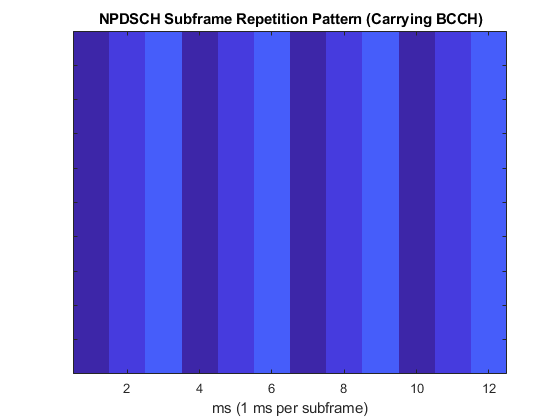% The NPDSCH repetition pattern for the current configuration is % displayed below displayPattern = false; % Display NPDSCH repetition pattern if displayPattern == true npdschInfo.displaySubframePattern; end 

### Block Error Rate Simulation Loop

This part of the example shows how to perform NB-IoT NPDSCH link level simulation and plot BLER results. The transmit and receive chain is depicted in the following figure.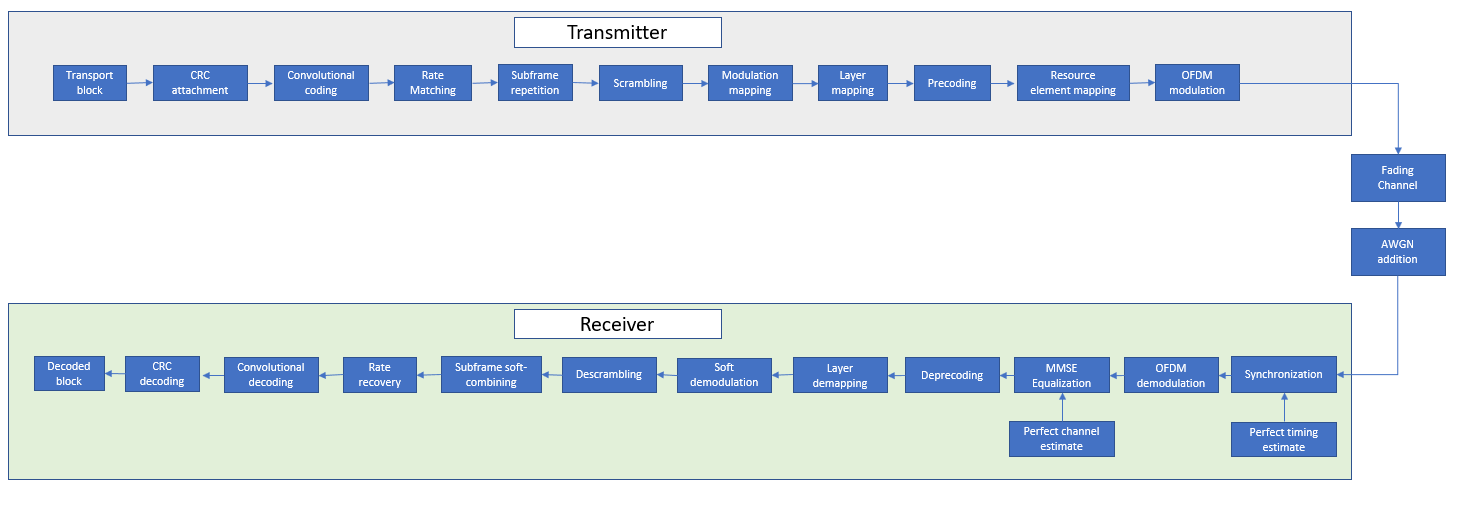A random stream of bits with the size of the desired transport block undergoes CRC encoding, convolutional encoding and rate matching to obtain the NPDSCH bits, which are repeated according to a specific subframe repetition pattern. Scrambling, modulation, layer mapping and precoding are then applied to form the complex NPDSCH symbols. These symbols along with the NRS signals are mapped to the grid and OFDM modulated to create the time domain waveform. This is then passed through a fading channel and AWGN is added. The noisy waveform is then synchronized and demodulated. Channel estimation and equalization is performed on the recovered NPDSCH symbols after which channel decoding and demodulation are performed to recover the transport block. After de-scrambling, the repetitive subframes are soft-combined before rate recover. The transport block error rate is calculated for each SNR point. The evaluation of the block error rate is based on the assumption that all the subframes in a bundle is used to decode the transport block at the UE. A bundle is defined in the MAC layer (see 3GPP TS 36.321 5.3.2.1 [ 3 ]) as the npdsch.NSFnpdsch.NRep subframes used to carry a transport block.

 % Absolute subframe number at the starting point of the simulation NSubframe = enb.NFrame*10+enb.NSubframe; % Initialize BLER and throughput result maxThroughput = zeros(length(SNRdB),1); simThroughput = zeros(length(SNRdB),1); bler = zeros(1,numel(SNRdB)); % The temporary variables 'enb_init' and 'channel_init' are used to create % the temporary variable 'enb' and 'channel' within the SNR loop to create % independent simulation loops for the 'parfor' loop enb_init = enb; channel_init = channel; for snrIdx = 1:numel(SNRdB) % parfor snrIdx = 1:numel(SNRdB) % To enable the use of parallel computing for increased speed comment out % the 'for' statement above and uncomment the 'parfor' statement below. % This needs the Parallel Computing Toolbox. If this is not installed % 'parfor' will default to the normal 'for' statement. % Set the random number generator seed depending to the loop variable % to ensure independent random streams rng(snrIdx,'combRecursive'); fprintf('\nSimulating %d transport blocks at %gdB SNR\n',numTrBlks,SNRdB(snrIdx)); enb = enb_init; % Initialize eNodeB configuration channel = channel_init; % Initialize fading channel configuration txcw = []; % Initialize the transmitted codeword numBlkErrors = 0; % Number of transport blocks with errors estate = []; % Initialize NPDSCH encoder state dstate = []; % Initialize NPDSCH decoder state lastOffset = 0; % Initialize overall frame timing offset offset = 0; % Initialize frame timing offset subframeGrid = zeros(12,14,enb.NBRefP); % Initialize the slot grid subframeIdx = NSubframe; numRxTrBlks = 0; while (numRxTrBlks < numTrBlks) % Set current subframe and frame numbers enb.NSubframe = mod(subframeIdx,10); enb.NFrame = floor((subframeIdx)/10); % Generate the NPSS symbols and indices npssSymbols = lteNPSS(enb); npssIndices = lteNPSSIndices(enb); % Map the symbols to the subframe grid subframeGrid(npssIndices) = npssSymbols; % Generate the NSSS symbols and indices nsssSymbols = lteNSSS(enb); nsssIndices = lteNSSSIndices(enb); % Map the symbols to the subframe grid subframeGrid(nsssIndices) = nsssSymbols; % Establish if either NPSS or NSSS is transmitted and if so, % do not transmit NPDSCH in this subframe isDataSubframe = isempty(npssSymbols) && isempty(nsssSymbols); % Create a new transport block and encode it when the % transmitted codeword is empty. The receiver sets the codeword % to empty to signal that all subframes in a bundle have been % received (it is also empty before the first transmission) if isempty(txcw) txTrBlk = randi([0 1],trblklen,1); txcw = lteNDLSCH(rmoutlen,txTrBlk); end if (isDataSubframe) % Generate NPDSCH symbols and indices for a subframe [txNpdschSymbols,estate] = lteNPDSCH(enb,npdsch,txcw,estate); npdschIndices = lteNPDSCHIndices(enb,npdsch); % Map the symbols to the subframe grid subframeGrid(npdschIndices) = txNpdschSymbols; % Generate the NRS symbols and indices nrsSymbols = lteNRS(enb); nrsIndices = lteNRSIndices(enb); % Map the symbols to the subframe grid subframeGrid(nrsIndices) = nrsSymbols; end % Perform OFDM modulation to generate the time domain waveform [txWaveform,ofdmInfo] = nbOFDMModulate(enb,subframeGrid); % Add 25 sample padding. This is to cover the range of delays % expected from channel modeling (a combination of % implementation delay and channel delay spread) txWaveform = [txWaveform; zeros(25, enb.NBRefP)]; %#ok<AGROW> % Initialize channel time for each subframe channel.InitTime = subframeIdx/1000; % Pass data through channel model channel.SamplingRate = ofdmInfo.SamplingRate; [rxWaveform,fadingInfo] = lteFadingChannel(channel, txWaveform); % Calculate noise gain including compensation for downlink power % allocation SNR = 10^(SNRdB(snrIdx)/20); % Normalize noise power to take account of sampling rate, which % is a function of the IFFT size used in OFDM modulation, and % the number of antennas N0 = 1/(sqrt(2.0*enb.NBRefP*double(ofdmInfo.Nfft))*SNR); % Create additive white Gaussian noise noise = N0*complex(randn(size(rxWaveform)), ... randn(size(rxWaveform))); % Add AWGN to the received time domain waveform rxWaveform = rxWaveform + noise; %------------------------------------------------------------------ % Receiver %------------------------------------------------------------------ % Perform timing synchronization, extract the appropriate % subframe of the received waveform, and perform OFDM % demodulation if(perfectChannelEstimator) offset = hPerfectTimingEstimate(fadingInfo); else % In this example, the subframe offset calculation relies % on NPSS present in subframe 5, so we need to pad the % subframes before it so that the frame offset returned by % lteNBDLFrameOffset is the offset for subframe 5 sfTsamples = ofdmInfo.SamplingRate*1e-3; if (enb.NSubframe==5) padding = zeros([sfTsamples*5,size(rxWaveform,2)]); offset = lteNBDLFrameOffset(enb, [padding; rxWaveform]); if (offset > 25) || (offset < 0) offset = lastOffset; end lastOffset = offset; end end % Synchronize the received waveform rxWaveform = rxWaveform(1+offset:end, :); % Perform OFDM demodulation on the received data to recreate the % resource grid rxSubframe = nbOFDMDemodulate(enb,rxWaveform); % Channel estimation if(perfectChannelEstimator) % Perfect channel estimation estChannelGrid = nbDLPerfectChannelEstimate(enb, channel, offset); noiseGrid = nbOFDMDemodulate(enb, noise(1+offset:end ,:)); noiseEst = var(noiseGrid(:)); else [estChannelGrid, noiseEst] = lteDLChannelEstimate( ... enb, cec, rxSubframe); end if (isDataSubframe) % Get NPDSCH indices npdschIndices = lteNPDSCHIndices(enb, npdsch); % Get PDSCH resource elements from the received subframe. Scale the % received subframe by the PDSCH power factor Rho. The PDSCH is % scaled by this amount, while the cell reference symbols used for % channel estimation (used in the PDSCH decoding stage) are not. [rxNpdschSymbols, npdschHest] = lteExtractResources(npdschIndices, ... rxSubframe, estChannelGrid); % Decode NPDSCH [rxcw,dstate,symbols] = lteNPDSCHDecode(... enb, npdsch, rxNpdschSymbols, npdschHest, noiseEst,dstate); % Decode the transport block when all the subframes in a bundle % have been received if dstate.EndOfTx [trblkout,blkerr] = lteNDLSCHDecode(trblklen,rxcw); numBlkErrors = numBlkErrors + blkerr; numRxTrBlks = numRxTrBlks + 1; % Re-initialize to enable the transmission of a new transport block txcw = []; end end subframeIdx = subframeIdx + 1; end % Calculate the block error rate bler(snrIdx) = numBlkErrors/numTrBlks; fprintf('NPDSCH BLER = %.4f \n',bler(snrIdx)); % Calculate the maximum and simulated throughput maxThroughput(snrIdx) = trblklen*numTrBlks; % Max possible throughput simThroughput(snrIdx) = trblklen*(numTrBlks-numBlkErrors); % Simulated throughput fprintf('NPDSCH Throughput(%%) = %.4f %%\n',simThroughput(snrIdx)*100/maxThroughput(snrIdx)); end 
Simulating 4 transport blocks at -32dB SNR 
Simulating 4 transport blocks at -32dB SNR NPDSCH BLER = 1.0000 NPDSCH Throughput(%) = 0.0000 % Simulating 4 transport blocks at -28dB SNR NPDSCH BLER = 1.0000 NPDSCH Throughput(%) = 0.0000 % Simulating 4 transport blocks at -24dB SNR NPDSCH BLER = 1.0000 NPDSCH Throughput(%) = 0.0000 % Simulating 4 transport blocks at -20dB SNR NPDSCH BLER = 1.0000 NPDSCH Throughput(%) = 0.0000 % Simulating 4 transport blocks at -16dB SNR NPDSCH BLER = 1.0000 NPDSCH Throughput(%) = 0.0000 % Simulating 4 transport blocks at -12dB SNR NPDSCH BLER = 0.2500 NPDSCH Throughput(%) = 75.0000 % Simulating 4 transport blocks at -8dB SNR NPDSCH BLER = 0.0000 NPDSCH Throughput(%) = 100.0000 % Simulating 4 transport blocks at -4dB SNR NPDSCH BLER = 0.0000 NPDSCH Throughput(%) = 100.0000 % Simulating 4 transport blocks at 0dB SNR NPDSCH BLER = 0.0000 NPDSCH Throughput(%) = 100.0000 % 
Simulating 4 transport blocks at -32dB SNR NPDSCH BLER = 1.0000 NPDSCH Throughput(%) = 0.0000 % Simulating 4 transport blocks at -28dB SNR NPDSCH BLER = 1.0000 NPDSCH Throughput(%) = 0.0000 % Simulating 4 transport blocks at -24dB SNR NPDSCH BLER = 0.2500 NPDSCH Throughput(%) = 75.0000 % Simulating 4 transport blocks at -20dB SNR NPDSCH BLER = 0.0000 NPDSCH Throughput(%) = 100.0000 % Simulating 4 transport blocks at -16dB SNR NPDSCH BLER = 0.0000 NPDSCH Throughput(%) = 100.0000 % Simulating 4 transport blocks at -12dB SNR NPDSCH BLER = 0.0000 NPDSCH Throughput(%) = 100.0000 % Simulating 4 transport blocks at -8dB SNR NPDSCH BLER = 0.0000 NPDSCH Throughput(%) = 100.0000 % Simulating 4 transport blocks at -4dB SNR NPDSCH BLER = 0.0000 NPDSCH Throughput(%) = 100.0000 % Simulating 4 transport blocks at 0dB SNR NPDSCH BLER = 0.0000 NPDSCH Throughput(%) = 100.0000 % 

### Plot Block Error Rate vs SNR results

 if repIdx == 1 fh = figure; grid on; hold on; xlabel('SNR (dB)'); ylabel('BLER'); legendstr = {['NRep = ' num2str(npdsch.NRep)]}; else legendstr = [legendstr ['NRep = ' num2str(npdsch.NRep)]]; %#ok<AGROW> end figure(fh); plot(SNRdB, bler, '-o');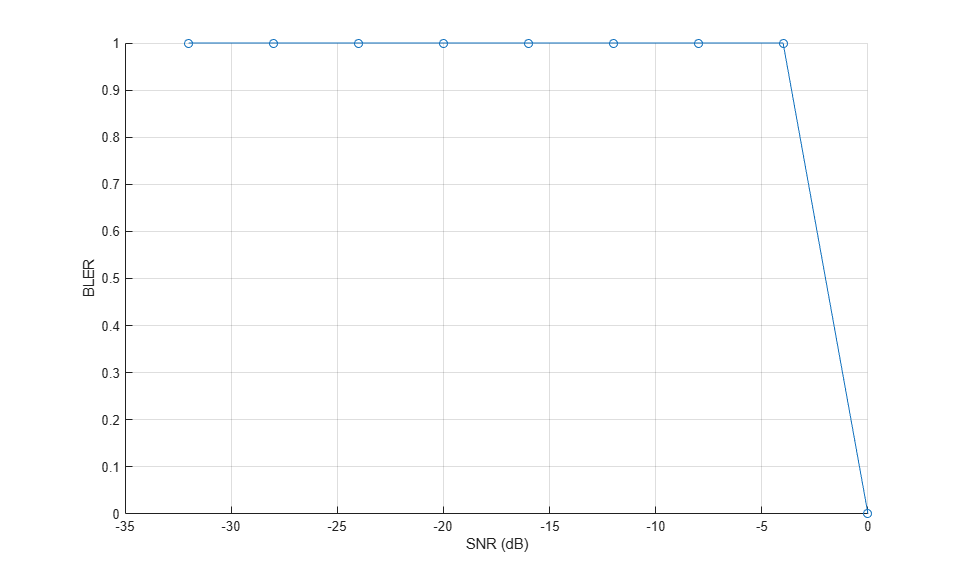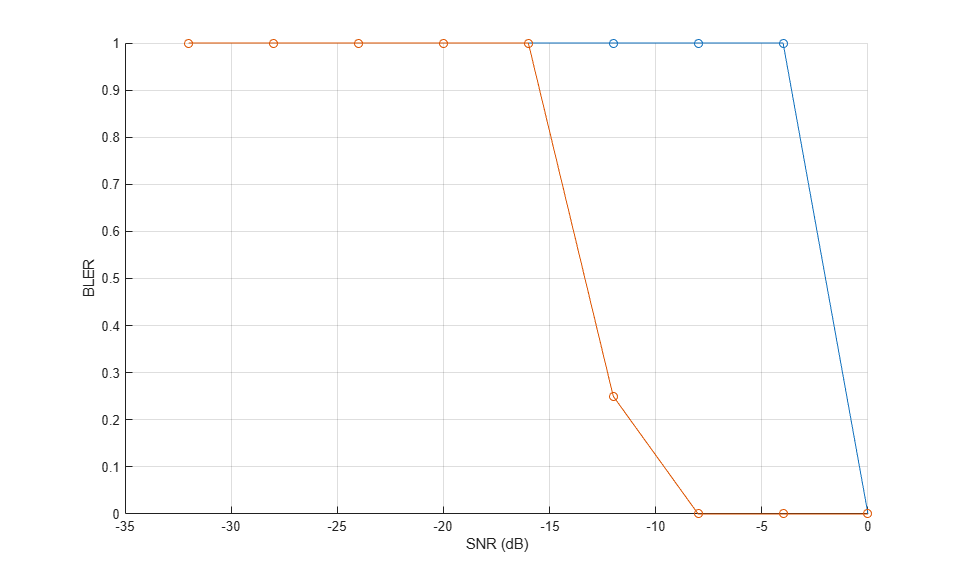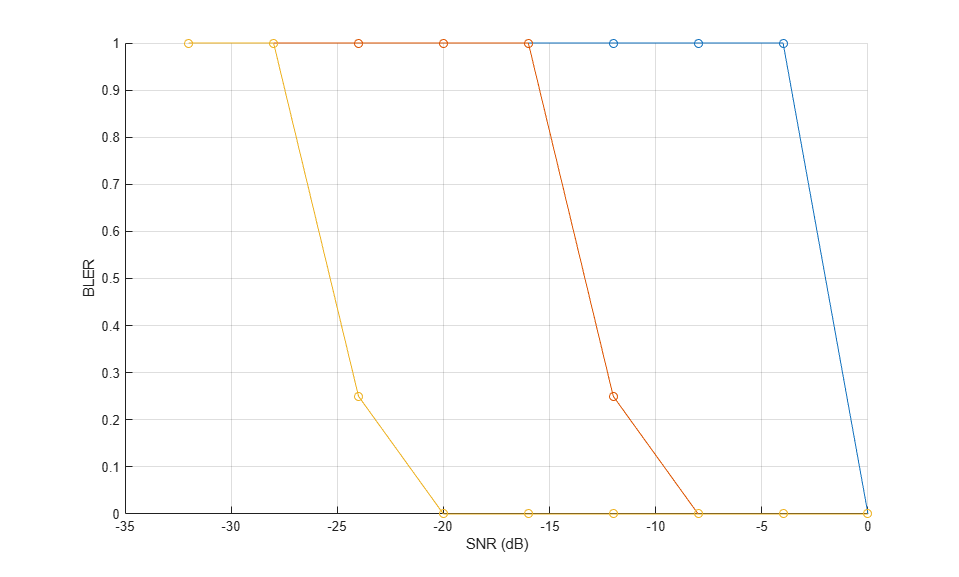end % Set figure title if strcmpi(NPDSCHDataType,'SIB1NB') npdsch.NSF = 8; end title([' ' char(npdsch.NPDSCHDataType) ': TBS=' num2str(trblklen)... '; NSF=' num2str(npdsch.NSF) '; ' num2str(enb_init.NBRefP) ' NRS port(s)' ]); legend(legendstr); 

The following plot shows the simulation run with numTrBlks set to 1000 while using the perfect channel estimator.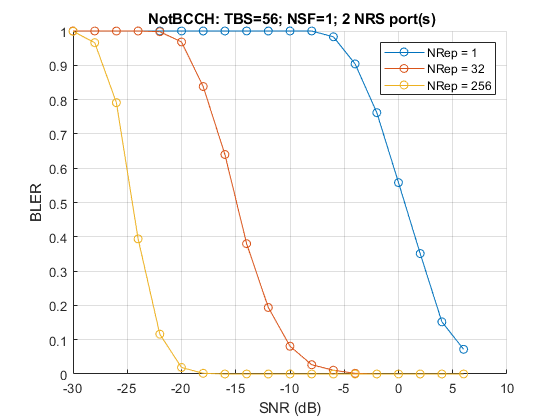### Appendix

This example uses the helper functions:

### Selected Bibliography

1. 3GPP TS 36.211 "Physical channels and modulation"

2. 3GPP TS 36.213 "Physical layer procedures"

3. 3GPP TS 36.321 "Medium Access Control (MAC) protocol specification"

4. 3GPP TS 36.101 "User Equipment (UE) radio transmission and reception"

### Local functions

% NB-IoT DL OFDM Modulator function [waveform,info] = nbOFDMModulate(enb,grid) % Apply default window size according to TS 36.104 Table E.5.1-1a if(~isfield(enb,'Windowing')) enb.Windowing = 6; end % Use NB-IoT SC-FDMA to get the 1/2 subcarrier shift on the OFDM modulation enb.NBULSubcarrierSpacing = '15kHz'; [waveform,info] = lteSCFDMAModulate(enb,grid); end % NB-IoT DL OFDM Demodulator function grid = nbOFDMDemodulate(enb,rxWaveform) % Use NB-IoT SC-FDMA to get the 1/2 subcarrier shift on the OFDM modulation enb.NBULSubcarrierSpacing = '15kHz'; grid = lteSCFDMADemodulate(enb,rxWaveform,0.55); % CP fraction of 0.55 end % NB-IoT DL Perfect Channel Estimator function H = nbDLPerfectChannelEstimate(enb,channel,timefreqoffset) % Reconfigure NB-IoT UL perfect channel estimator to perform DL perfect % channel estimation enb.NBULSubcarrierSpacing = '15kHz'; enb.NTxAnts = enb.NBRefP; enb.TotSlots = 2; H = lteULPerfectChannelEstimate(enb, channel,timefreqoffset); end 
NPDSCH BLER = 1.0000 NPDSCH Throughput(%) = 0.0000 % Simulating 4 transport blocks at -28dB SNR NPDSCH BLER = 1.0000 NPDSCH Throughput(%) = 0.0000 % Simulating 4 transport blocks at -24dB SNR NPDSCH BLER = 1.0000 NPDSCH Throughput(%) = 0.0000 % Simulating 4 transport blocks at -20dB SNR NPDSCH BLER = 1.0000 NPDSCH Throughput(%) = 0.0000 % Simulating 4 transport blocks at -16dB SNR NPDSCH BLER = 1.0000 NPDSCH Throughput(%) = 0.0000 % Simulating 4 transport blocks at -12dB SNR NPDSCH BLER = 1.0000 NPDSCH Throughput(%) = 0.0000 % Simulating 4 transport blocks at -8dB SNR NPDSCH BLER = 1.0000 NPDSCH Throughput(%) = 0.0000 % Simulating 4 transport blocks at -4dB SNR NPDSCH BLER = 1.0000 NPDSCH Throughput(%) = 0.0000 % Simulating 4 transport blocks at 0dB SNR NPDSCH BLER = 0.0000 NPDSCH Throughput(%) = 100.0000 %